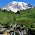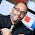## Friday, November 6, 2009

### Oversampling in General

Dear Data Miners,

I am trying to find out statistical reasons for balancing data sets when building models with binary targets, and nobody is able to intelligently describe why it is being done. In fact, there are mixed opinions on sampling when the response rate is low.

Based on literature and data mining professional opinions, here are few versions (assume that the response rate is 1%):

1) As long as the number of responders is approximately equal or greater than 10 times the number variables included, no additional sampling is needed.

2) Oversample or undersample (based on the total number of observations) at least until the response rate = 10%.

3) Oversample or undersample (based on the total number of observations) until the response rate = 50%.

4) Undersampling is useful only for cutting down on processing time; really no good reason to do it statistically as long as the number of observations for responders is "sufficient" (% does not matter).

Having an advanced degree in mathematics but not being a statistician, I would like to understand whether there really is any statistical benefit in doing that.

I appreciate your time answering this.

Sincerely,

Your fellow data miner

Many years ago, I was doing a churn model for SK Telecom (in South Korea) using SAS Enterprise Miner. A friend of mine at SAS, Anne Milley, had suggested that having a 50% density for a binary response model would produce optimal models. Her reasoning was that with a 50% density of each target value, the contrast between the two values would be maximized, making it easier to pick out patterns in the data.

I spent some time testing decision trees with all sorts of different densities. To my surprise, the decision trees with more than 30% density performed better than trees with lower densities, regardless of the splitting criterion and other factors. This convinced me that 50% is not a bad idea.

There is a reason why decision trees perform better on balanced samples. The standard pruning algorithm for decision trees uses classification as the metric for choosing subtrees. That is, a leaf chooses its dominant class -- the one in excess of 50% for two classes. This works best when the classes are evenly distributed in the data. (Why data mining software implementing trees doesn't take the original density into account is beyond me.)

In addition, the splitting criteria may be more sensitive to deviations around 50% than around other values.

Standard statistical techniques are insensitive to the original density of the data. So, a logistic regression run on oversampled data should produce essentially the same model as on the original data. It turns out that the confidence intervals on the coefficients do vary, but the model remains basically the same.

Hmmm, as I think about it, I wonder if the oversampling rate would affect stepwise or forward selection of variables. I could imagine that, when testing each variable, the variance in results using a rare target would be larger than the variance using a balanced model set. This, in turn, might lead to a poorer choice of variables. But I don't know if this is the case.

For neural networks, the situation is more complicated. Oversampling does not necessarily improve the neural network -- there is no theoretical reason why. However, it does allow the network to run on a smaller set of data, which makes convergence faster. This, in turn, allows the modeler to experiment with different models. Faster convergence is a benefit in other ways.

Some other techniques such as k-means clustering and nearest neighbor approaches probably do benefit from oversampling. However, I have not investigated these situations in detail.

Because I am quite fond of decision trees, I prefer a simple rule, such as "oversample to 50%", since this works under the maximum number of circumstances.

In response to your specific questions, I don't think that 10% is a sufficient density. If you are going to oversample, you might as well go to 50% -- there is at least an elegant reason why (the contrast idea between the two response values). If you don't have enough data, then use weights instead of oversampling to get the same effect.

In the end, though, if you have the data and you have the software, try out different oversampling rates and see what produces the best models!

#### 5 comments:

1.Hi Gordon,

About oversampling and regression: if I'm being a bad puppy and using stepwise/forwards/backwards with a lot of variables, and I have an unbalanced data set (say around 1% positive) then in my experience running the process as-is produces much less satisfactory results than using an oversampled data set.

2.Great discussion of oversampling and some of the potential benefits it can bring. I find it interesting that you discuss whether or not to oversample strictly in terms of the algorithm chosen, which I think is likely right on, but it makes me wonder about two things.

First, is the decision to oversample a function only of the algorithm, or is it also a function of some of the features of the dataset as well. If so, I wonder if some of the features that have an impact might be more than just the number of instances per class, but maybe other characteristics of the data such as skewness or entropy, or something else that might not be immediately obvious.

Second, if the characteristics of dataset don’t have much of an impact (e.g., maybe they make a difference, but if it turns out to be so small compared to the difference of the algorithms), then I wonder if it would be better for the algorithms themselves to take care of, and take into account, the oversampling. That way they could each account for how this affects their respective inductive biases.

3.Popular statistical procedures, like logistic regression, can sharply underestimate the probability of rare events (http://nrs.harvard.edu/urn-3:HUL.InstRepos:4125045). Thus, oversampling helps to correct for this bias (http://www.dnbtransunion.com/insights/whitepapers/whitepaper_event.pdf)

1.The second link does not work any more. Could you specify the title and author of the article? Thank you!

2.I tried to track down the paper that Joey C referenced, but he did not leave enough detail.

Your comment will appear when it has been reviewed by the moderators.